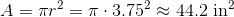High School Math : Geometry

Example Questions

Example Question #16 : How To Find The Area Of A Circle

A manufacturer makes wooden circles out of square blocks of wood. If the wood costs \$0.20 per square inch, what is the minimum waste cost possible for cutting a circle with a radius of 25 in.?

500 dollars

625 dollars

625 - 25π dollars

2500 - 625π dollars

500 - 125π dollars

500 - 125π dollars

Explanation:

The smallest block from which a circle could be made would be a square that perfectly matches the diameter of the given circle. (This is presuming we have perfectly calibrated equipment.) Such a square would have dimensions equal to the diameter of the circle, meaning it would have sides of 50 inches for our problem. Its total area would be 50 * 50 or 2500 in2.

Now, the waste amount would be the "corners" remaining after the circle was cut. The area of the circle is πr2 or π * 252 = 625π in2. Therefore, the area remaining would be 2500 - 625π. The cost of the waste would be 0.2 * (2500 – 625π). This is not an option for our answers, so let us simplify a bit. We can factor out a common 5 from our subtraction. This would give us: 0.2 * 5 * (500 – 125π). Since 0.2 is equal to 1/5, 0.2 * 625 = 125. Therefore, our final answer is: 500 – 125π dollars.

Example Question #52 : Circles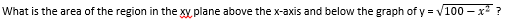50π

25π

100π

10π

20π

50π

Explanation: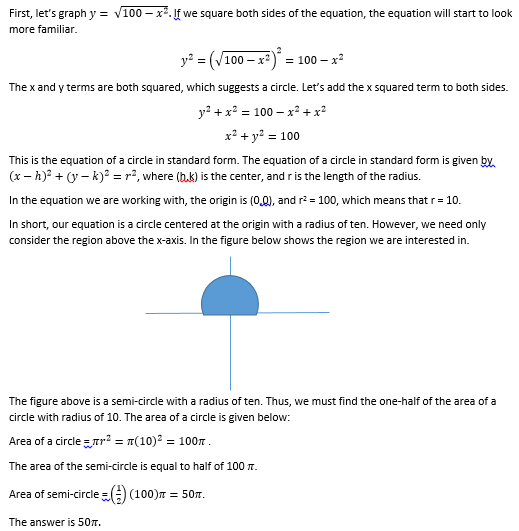Example Question #18 : How To Find The Area Of A Circle

A circle with diameter of length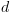is inscribed in a square. Which of the following is equivalent to the area inside of the square, but outside of the circle?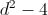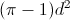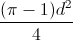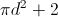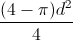Explanation:

In order to find the area that is inside the square but outside the circle, we will need to subtract the area of the circle from the area of the square. The area of a circle is equal to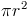. However, since we are given the length of the diameter, we will need to solve for the radius in terms of the diameter. Because the diameter of a circle is twice the length of its radius, we can write the following equation and solve for: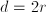Divide both sides by 2.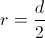We will now substitute this into the formula for the area of the circle.

area of circle =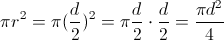We next will need to find the area of the square. Because the circle is inscribed in the square, the diameter of the circle is equal to the length of the circle's side. In other words, the square has side lengths equal to d. The area of any square is equal to the square of its side length. Therefore, the area of the square is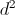.

area of square =Lastly, we will subtract the area of the circle from the area of the square.

difference in areas =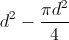We will rewriteso that its denominator is 4.

difference in areas =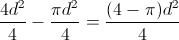The answer is.

Example Question #19 : How To Find The Area Of A Circle

An original circle has an area of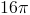. If the radius is increased by a factor of 3, what is the ratio of the new area to the old area?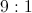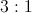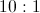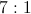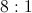Explanation:

The formula for the area of a circle is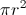. If we increase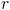by a factor of 3, we will increase the area by a factor of 9.

Example Question #391 : Psat Mathematics

A square has an area of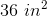.  If the side of the the square is the same as the diameter of a circle, what is the area of the circle?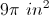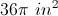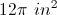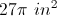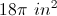Explanation:

The area of a square is given by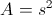so we know that the side of the square is 6 in.  If a circle has a diameter of 6 in, then the radius is 3 in.  So the area of the circle is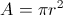or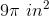.

Example Question #81 : Geometry

Mary has a decorative plate with a diameter of ten inches. She places the plate on a rectangular placemat with a length of 18 inches and a width of 12 inches. How much of the placemat is visible?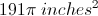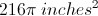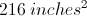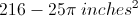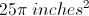Explanation:

First we will calculate the total area of the placemat: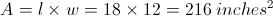Next we will calculate the area of the circular placeAnd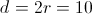So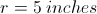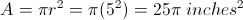We will subtract the area of the plate from the total areaExample Question #82 : Geometry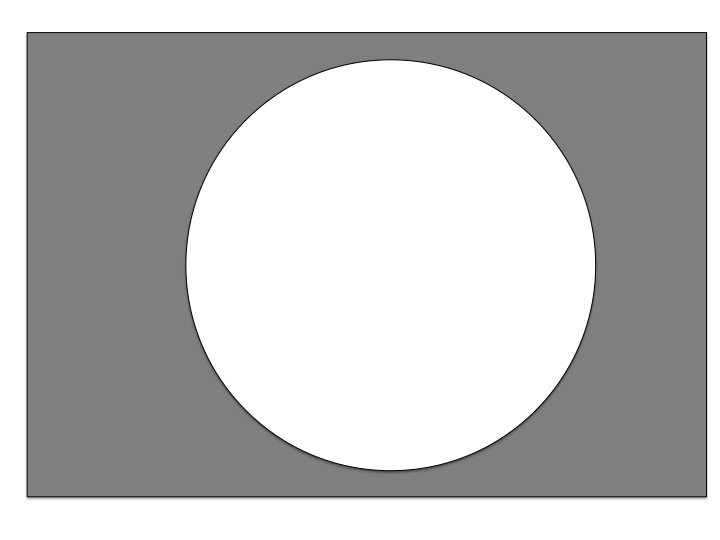The picture above contains both a circle with diameter 4, and a rectangle with length 8 and width 5. Find the area of the shaded region. Round your answer to the nearest integer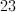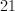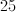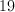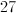Explanation:

First, recall that the diameter of a circle is twice the value of the radius. Therefore a circle with diameter 4 has a radius of 2. Next recall that the area of a circle with radius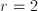is: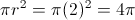The area of the rectangle is the length times the width: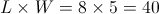The area of the shaded region is the difference between the 2 areas: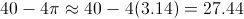The nearest integer is 27.

Example Question #83 : Geometry

Allen was running around the park when he lost his keys.  He was running around aimlessly for the past 30 minutes.  When he checked 10 minutes ago, he still had his keys.  Allen guesses that he has been running at about 3m/s.

If Allen can check 1 square kilometer per hour, what is the longest it will take him to find his keys?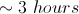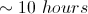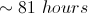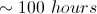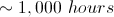Explanation:

Allen has been running for 10 minutes since he lost his keys at 3m/s.  This gives us a maximum distance of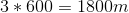from his current location.  If we move 1800m in all directions, this gives us a circle with radius of 1800m.  The area of this circle is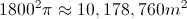Our answer, however, is asked for in kilometers.  1800m=1.8km, so our actual area will be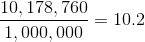square kilometers.  Since he can search 1 per hour, it will take him at most 10.2 hours to find his keys.

Example Question #84 : Geometry

To the nearest tenth, give the diameter of a circle with area 100 square inches.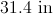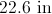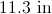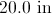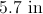Explanation:

The relationship between the radius and the area of a circle can be given as.

We can substitute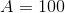and solve for: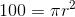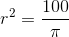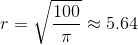Double this to get the diameter: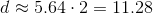, which we round to 11.3.

Example Question #85 : Geometry

To the nearest tenth, give the area of a circle with diameter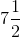inches.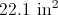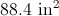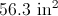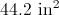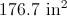The radius of a circle with diameterinches is half that, or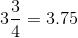inches. The area of the circle is